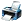# Arithmetic question

721 viewsposted Nov 1, 2016

+1 voteanswer Nov 4, 2016

above ans is correct i also solve it ans is 6answer Dec 2, 2016

The above ans is correct .answer May 25, 2017 by

Let us make it more clear :
Ans. a/(b+c+d)+1+b/(c+d+e)+1+c/(d+a+b)+1+d/(a+b+c)+1-4
=(a+b+c+d){1/(b+c+d)........}-4=6×5/3 -4=10-4=6 .answer Jun 15, 2017 by

Hello,
If you are preparing for Arithmetic
you can prepare from the following books
ML KHANNA
IA MARRON for basic concepts
Hall knight for Higher Algebra
Also, you must check out for JEE Advanced Eligibility 2018answer Sep 5, 2017

Hello,
You have asked an important question from the Arithmetic part in mathematics,as this is the most important topic from the JEE Point of View
As the JEE Advanced 2018 Date is out
The Arithmetic part covers good weight age in JEE Exams
The JEE Advanced date is out
Is going to be conducted on 20th May.answer Sep 21, 2017
Similar Questions